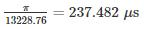Courses

# Test: Power Electronics - 1

## 10 Questions MCQ Test Mock Test Series for Electrical Engineering (EE) GATE 2020 | Test: Power Electronics - 1

Description
This mock test of Test: Power Electronics - 1 for Electrical Engineering (EE) helps you for every Electrical Engineering (EE) entrance exam. This contains 10 Multiple Choice Questions for Electrical Engineering (EE) Test: Power Electronics - 1 (mcq) to study with solutions a complete question bank. The solved questions answers in this Test: Power Electronics - 1 quiz give you a good mix of easy questions and tough questions. Electrical Engineering (EE) students definitely take this Test: Power Electronics - 1 exercise for a better result in the exam. You can find other Test: Power Electronics - 1 extra questions, long questions & short questions for Electrical Engineering (EE) on EduRev as well by searching above.
QUESTION: 1

### A power diode is in the forward conduction mode and the forward current is now decreased. The reverse recovery time of the diode is trr and the rate of fall of the diode current is di/dt. What is the stored charge?

Solution:

The reverse recovery characteristic of a power diode is shown below. reverse recovery time trr composed of ta and tb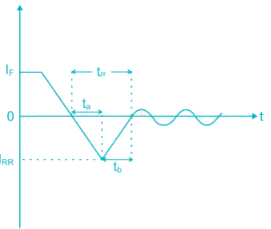ta is the time between zero crossings and peak reverse current IRR and tb is measured from reverse peak IRR value to 0.25 IRR.

If the characteristics are assumed to be triangular (i.e. abrupt recovery) then from the figure charge stored is

QRR = area of the triangle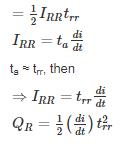QUESTION: 2

### A G.T.O with anode fingers has

Solution: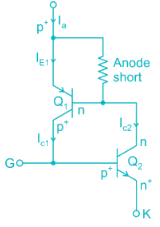The short circuiting fingers, also called anode-shorts, leads to short-circuit of the emitter p+(Anode A) with base n of Q1 transistor as shown in Fig. For anode or emitter current Ia, effective emitter current IE1 is reduced because of anode short. This further decreases collector current IC1. Therefore, effective current gain α1 of Q1, now given by IC1/Ia gets reduced. So by anode-short structure, α1 is reduced but α2 remains unchanged as desired.

Anode-short, however, reduces reverse voltage blocking capability. With reverse biased GTO, junction that blocks reverse voltage is J3 only. Junction J1 blocks no voltage because of n+fingers in between p+ anode. As J3 junction has large doped layers p+, n+ on its two sides, J3 has lower breakdown voltage, of the order of about 20 to 30 V.

QUESTION: 3

### Four power semiconductor device are shown in the figure along with relevant terminals the device that can carry dc current continuously in the Direction shown when gated appropriately is (are)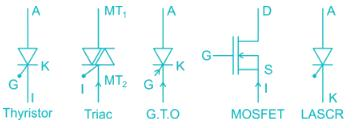Solution:
• Thyristor is unidirectional and bipolar switch. It carry current Anode to cathod and block the current cathode to anode
• Triac conduct in both the direction A triac is thus a bidirectional thyristor with three terminal
• GTO is just like SR it is unidirectional and bipolar It conducts only Anode to cathode
• MOSFET is bidirectional but unipolar device
• LASER is conduct only one direction.
*Answer can only contain numeric values
QUESTION: 4

In power transistor, during turn on process voltage and current change linearly from 400 V and 0 V and 0 A to 300 A respectively in 3 μS. the energy loss during turn on process in given by _____Joule (up to 2 decimal places)

Solution:

Concept: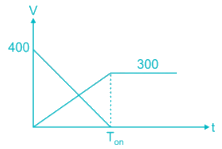Change in V and L are shown below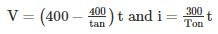Energy loss during turn on process =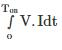Calculations: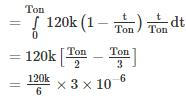= 20 × 103 × 3 × 10-6

= 0.06 J

*Answer can only contain numeric values
QUESTION: 5

A power transistor has its switching waveforms as shown in fig. If the average power loss in the transistor is limited to 470 W, find the switching frequency at which this transistor can be operated – (in kHz)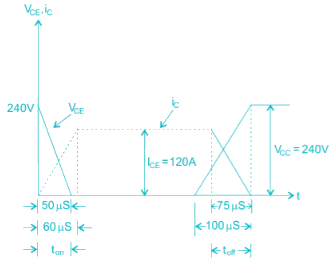Solution:

Energy loss during turn – on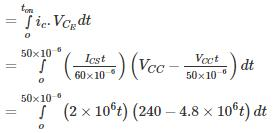= 0.2 watt – sec.

Energy loss during turn – off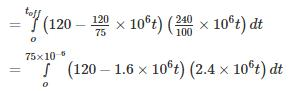= 0.27 watt – sec

Total energy loss in one cycle = 0.2 + 0.27

= 0.47 watt – sec.

Average power loss in transistor

= switching frequency × energy loss in one cycle

⇒ Switching frequency =470/0.47

= 1000 Hz.

QUESTION: 6

For a power transistor typical switching waveform shown in Fig. the various parameter of the transistor circuit are as under: Vcc = 220V, VCES = 2V, Ics = 80 A, td = 0.4 μs tr = 1 μs f = 5 kHz and ICEO = 2mA. Determine Average Power loss during turn-on time.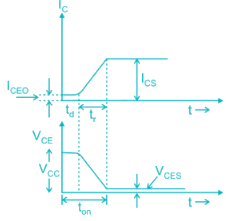Solution:

Concept:

Average power loss during delay time with o < t < td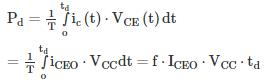Average power loss during rise time is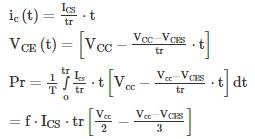Calculations:

Pd = 5 × 103 × 2 × 10−3 × 220 × 4 × 10−6 = 88mW

Pr = 5 × 103 × 80 × 1 × 10−6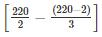= 14.933W
Total power loss during turn on time = 14.933 + 0.00088 = 14.9339 W

QUESTION: 7

In a power electronics circuit, MOSFET is using as the switch, MOSFET with an ON state resistance 50 mΩ has with the following switching characteristics. The power losses associated with MOSFET are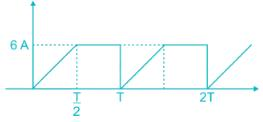Solution:

Concept: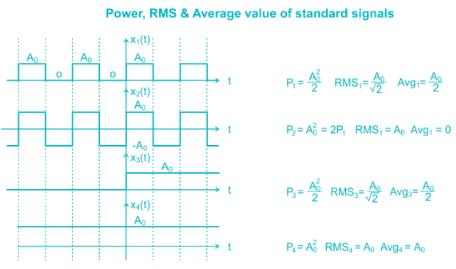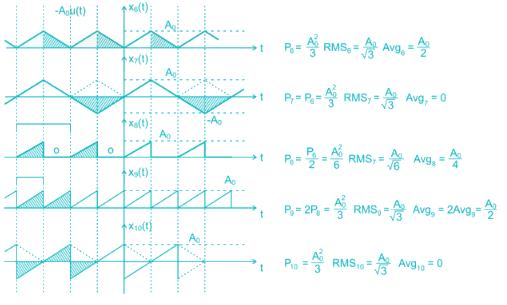Calculations: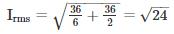P = Irms2 × 50 × 10-3 = 24 × 50 × 10-3 = 1.2 W

*Answer can only contain numeric values
QUESTION: 8

Find the time required (in hour) to deliver a charge of 400 Ah through a single phase half-wave diode Rectifier with an output current 200 A rms and with sinusoidal input voltage. Assume diode conduction over a half-cycle

Solution:

Concept:

For half-wave diode rectifier

R.M.S value of the output voltage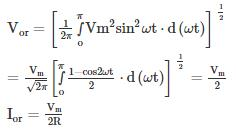Average value of load current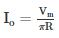Calculation: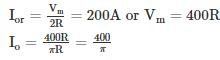Io × time = 400 Ah
∴ Time requried =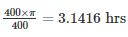QUESTION: 9

A gate turn off (GTO) thyristor has capacity to

Solution: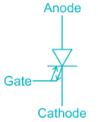• The Gate turn off thyristor (GTO) is a four-layer PNPN power semiconductor switching device that can be turned on by a short pulse of gate current and
• can be turned off by a reverse gate pulse.
• The magnitude of latching, holding currents is more. The latching current of the GTO is several times more as compared to conventional thyristors of the
• same rating.
• On state voltage drop and the associated loss is more.
• Due to the multi-cathode structure of GTO, triggering gate current is higher than that required for normal SCR.
• Gate drive circuit losses are more. Its reverse voltage blocking capability is less than the forward voltage blocking capability.
• GTO has the capability of being turned off by a negative gate – current pulse
*Answer can only contain numeric values
QUESTION: 10

For the circuit shown in Fig R = 10 Ω L = 1 mH C = 5 μF and VS = 230 V. the circuit is initially relaxed determine conduction time of diode _________ μs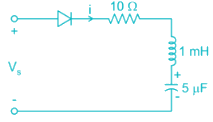Solution:

Concept:

Apply KVL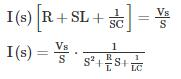The roots of the characteristic equation are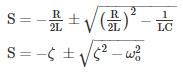Ringing Frequency in rod/sec = ω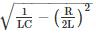Calculations:

Conduction time of diode = π/ωr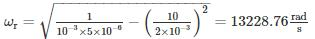Conduction time =